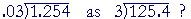DIVIDE DECIMALS

PROBLEMS

Do the problem yourself first!

1.   What property of division allows us to rewriteIf the divisor and the dividend are multiplied by the same number, the quotient will not change.

Lesson 11, Property 2.

2.   Make each divisor a whole number.  Calculate the quotient.

 __ __ __ __ a) .03)24 b) .006)18 c) .5)12 d) .0008)3 800 3000 24 3750
 ___ __ ___ ___ e) .04)115 f) .006)51 g) .9)126 h) .0025)11 2875 8500 140 4400

3.  Make each divisor a whole number. Calculate the quotient.

 ____ _____ _____ ______ a) .6)4.26 b) .03).2052 c) .005).0655 d) .009).02466 7.1 6.84 13.1 2.74
 _____ ______ ______ ______ e) .08).0032 f) .02).00018 g) .4)24.016 h) .012).01296 .04 .009 60.04 1.08
 ____ ____ ___ ____ i) .6)418.2 j) .0012).732 k) .07)2.8 l) .0016)12.8 697 610 40 8000

4.  Distinguish between the dividend and the divisor, and find the
4.  quotient.

4.  a)  .0005 ÷ .05 = .01             b)  .05 ÷ .0005 = 100

5.  c)  1.6 ÷ 16 = .1                 d)  16 ÷ 1.6 = 10

5.  a)  What number times 5 is 44?   44 ÷ 5 = 8.8

5.  b)  What number times .08 is .004?   .004 ÷ .08 = .05

Continue on to the next Lesson.

or